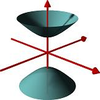#### You may also like### A Close Match

Can you massage the parameters of these curves to make them match as closely as possible?### Prime Counter

A short challenge concerning prime numbers.### The Right Volume

Can you rotate a curve to make a volume of 1?

# Happy birthDay

##### Age 16 to 18Challenge Level
Zeller worked out a formula to calculate the day of the week for any date.
$$d= \left(n+\mbox{int}\left[\frac{(m+1)\times 26}{10}\right]+y+\mbox{int}\left[\frac{y}{4}\right]+\mbox{int}\left[\frac{C}{4}\right]-2C\right)\mbox{mod }7$$
This formula contains a lots of symbols and terminology, as follows:

In Zeller's formula $d$ stands for the day of the week, numbered $0$ to $6$ for Saturday - Friday.

$n$ is the day of the month, numbered 1 to 28, 29, 30 or 31 depending on the month in question.

$m$ is the month, numbered from $3-12$ for March - December; $m=13$ in January and $m=14$ in February.

$y$ is the last two digits of the year, or the last two digits of the previous year if $m=13$ or $14$.

$C$ is the first two digits of the year.

'int[$x$]' is a function which rounds $x$ down to the nearest integer or returns $x$ if $x$ is already an integer.

'mod 7' means return the remainder when divided by 7.

1) Check that you can use the formula to work out the day of the week for a few dates.

2) (Difficult extension) Work out why it is, either roughly or exactly, that Zeller's algorithm works. A spreadsheet might help to work out why each term is present in the algorithm.

Did you know ... ?

Date algorithms are complicated by leap years, when there are 29 days in February. Leap years occur roughly every 4 years, although on occasions a leap year is skipped. The precise rule for determining leap years is as follows:

If the year modulo 400 is 0 then it is a leap year; else if the year modulo 100 is 0 then it is not a leap year;  else if the year modulo 4 is 0 then it is a leap year; else it is not a leap year.

Some human calculators are able to run Zeller's algorithm in their heads. Can you learn how to do this? You can see an awesome human calculator in action on You Tube.